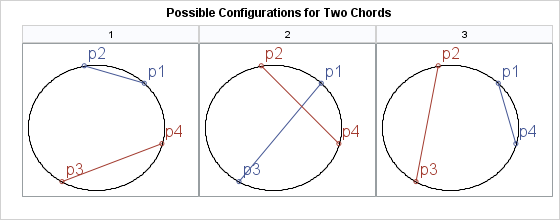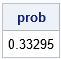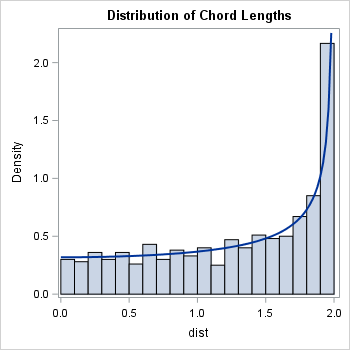In a previous article, I showed how to find the intersection (if it exists) between two line segments in the plane. There are some fun problems in probability theory that involve intersections of line segments. One is "What is the probability that two randomly chosen chords of a circle intersect?" This article shows how to create a simulation in SAS to estimate the probability.

For this problem, a "random chord" is defined as the line segment that joins two points chosen at random (with uniform probability) on the circle. The probability that two random chords intersect can be derived by using a simple counting argument. Suppose that you pick four points at random on the circle. Label the points according to their polar angle as p1, p2, p3, and p4. As illustrated by the following graphic, the points are arranged on the circle in one of the following three ways. Consequently, the probability that two random chords intersect is 1/3 because the chords intersect in only one of the three possible arrangements.A simulation in SAS

You can create a simulation to estimate the probability that two random chords intersect. The intersection of two segments can be detected by using either of the two SAS/IML modules in my article about the intersection of line segments. The following simulation generates four angles chosen uniformly at random in the interval (0, 2π). It converts those points to (x,y) coordinates on the unit circle. It then computes whether the chord between the first two points intersects the chord between the third and fourth points. It repeats this process 100,000 times and reports the proportion of times that the chords intersect.

 proc iml; /* Find the intersection between 2D line segments [p1,p2] and [q1,q2]. This function assumes that the line segments have different slopes (A is nonsingular) */ start IntersectSegsSimple(p1, p2, q1, q2); b = colvec(q1 - p1); A = colvec(p2-p1) || colvec(q1-q2); /* nonsingular when segments have different slopes */ x = solve(A, b); /* x = (s,t) */ if all(0<=x && x<=1) then /* if x is in [0,1] x [0,1] */ return (1-x)*p1 + x*p2; /* return intersection */ else /* otherwise, segments do not intersect */ return ({. .}); /* return missing values */ finish;   /* Generate two random chords on the unit circle. Simulate the probability that they intersect */ N = 1e5; theta = j(N, 4); call randseed(123456); call randgen(theta, "uniform", 0, 2*constant('pi')); intersect = j(N,1,0); do i = 1 to N; t = theta[i,]`; /* 4 random U(0, 2*pi) */ pts = cos(t) || sin(t); /* 4 pts on unit circle */ p1 = pts[1,]; p2 = pts[2,]; q1 = pts[3,]; q2 = pts[4,]; intersect[i] = all(IntersectSegsSimple(p1, p2, q1, q2) ^= .); end;   prob = mean(intersect); print prob;This simulation produces an estimate that is close to the exact probability of 1/3.